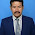### Tugas

IMPLEMENTATION OF QUANTITATIVE METHODS IN PSYCHOLOGY OF MATHEMATICS EDUCATION
Quantitative methods are research techniques that are used to gather quantitative data - information dealing with numbers and anything that is measurable. The other definition of quantitative methods are research method that relies less on interviews, observations, small numbers of questionnaires, focus groups, subjective reports and case studies but is much more focused on the collection and analysis of numerical data and statistics. It means that in process data by quantitative methods, data must be shaped as numeric data. We can use quantitative methods to process data that’s interrelated with psychology of mathematics education in the school.
A kind of quantitative methods is inferential statistics. Inferential statistics means procedure used to estimate parameters (characteristics of populations) from statistics (characteristics of samples).
In psychology of mathematics education, data that can process with inferential statistic can be value of student’s test or data from teacher’s research. Teacher can do the observation to the students about student’s work. For example, in the class teacher giving test for the students. The test can be mathematics problem from the material from the chapter that’s having learned by the students.
In the implementation of the test, teacher can look at the students. Look for student’s behavior in doing the test. With looking to several students teacher’s can know readiness of students and can conclude that students have study or not. If several of them look confusion it means that they haven’t study before. If they look relax, it means that they have study before.
In the mean time, the value of the test given can showed the result of student’s ability. Teacher can only look for several of tests value. If from several value, there are good values, teacher can concluded that students have study before, they understand with this chapter and they ready to learn the next chapter. If from several value, there are bad values, teacher can concluded that students not study before, they not understand with this chapter, and they unready to learn more. Good and bad value here, means the students value is above or below from standard value.
From the example above we can show that from several worksheet, we can presume the readiness all of the students. It is an example of implementation of inferential statistics in mathematics education. Students psychology can show by their behavior in doing test and from several values can know condition of students whether all of them ready or not without looking for all of worksheet.
REFERENCES:
http://en.wikipedia.org/wiki/Quantitative_methods
1.